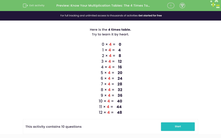# Know Your Multiplication Tables: The 4 Times Table

In this worksheet, students must recall multiplication facts from the 4 times table.Key stage:  KS 2

Curriculum topic:   Number: Multiplication and Division

Curriculum subtopic:   Use Multiplication/Division Facts (3, 4 and 8)

Popular topics:   Times Table worksheets

Difficulty level:#### Worksheet Overview

In this activity, we will be multiplying numbers by 4.

Here is the 4 times table.

Try to learn it by heart.

0 × 4 =   0

1 × 4 =   4

2 × 4 =   8

3 × 4 =   12

4 × 4 =   16

5 × 4 =   20

6 × 4 =   24

7 × 4 =   28

8 × 4 =   32

9 × 4 =   36

10 × 4 =   40

11 × 4 =   44

12 × 4 =   48

To multiply by 4, skip counting is a good technique.

4, 8, 12, 16, 20, 24, 28, 32, 36, 40, 44, 48

If you are stuck, choose a fact you know well to count on or back from.

So, we know that 10 × 4 = 40

Count back 4 to find 9 × 4

40, 39, 38, 37, 36

Count on 4 to find 11 × 4

40, 41, 42, 43, 44

Let's look at an example

Example

Find 5 × 4.

To find 5 lots of 4, we can skip count and choose the 5th multiple.

4, 8, 12, 16, 20

5 × 4 = 20

Now you're ready to try some questions. Good luck!

### What is EdPlace?

We're your National Curriculum aligned online education content provider helping each child succeed in English, maths and science from year 1 to GCSE. With an EdPlace account you’ll be able to track and measure progress, helping each child achieve their best. We build confidence and attainment by personalising each child’s learning at a level that suits them.

Get started#### Popular Maths topics

••••